Ex 14.3

Chapter 14 Class 10 Statistics
Serial order wise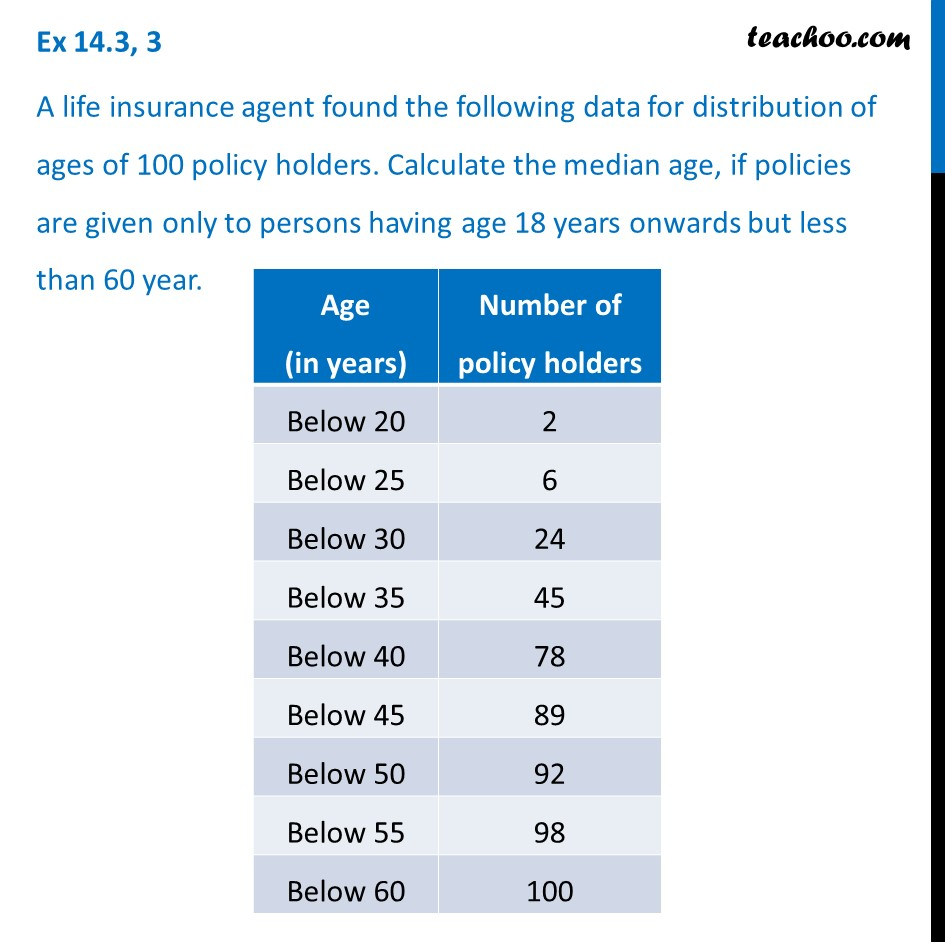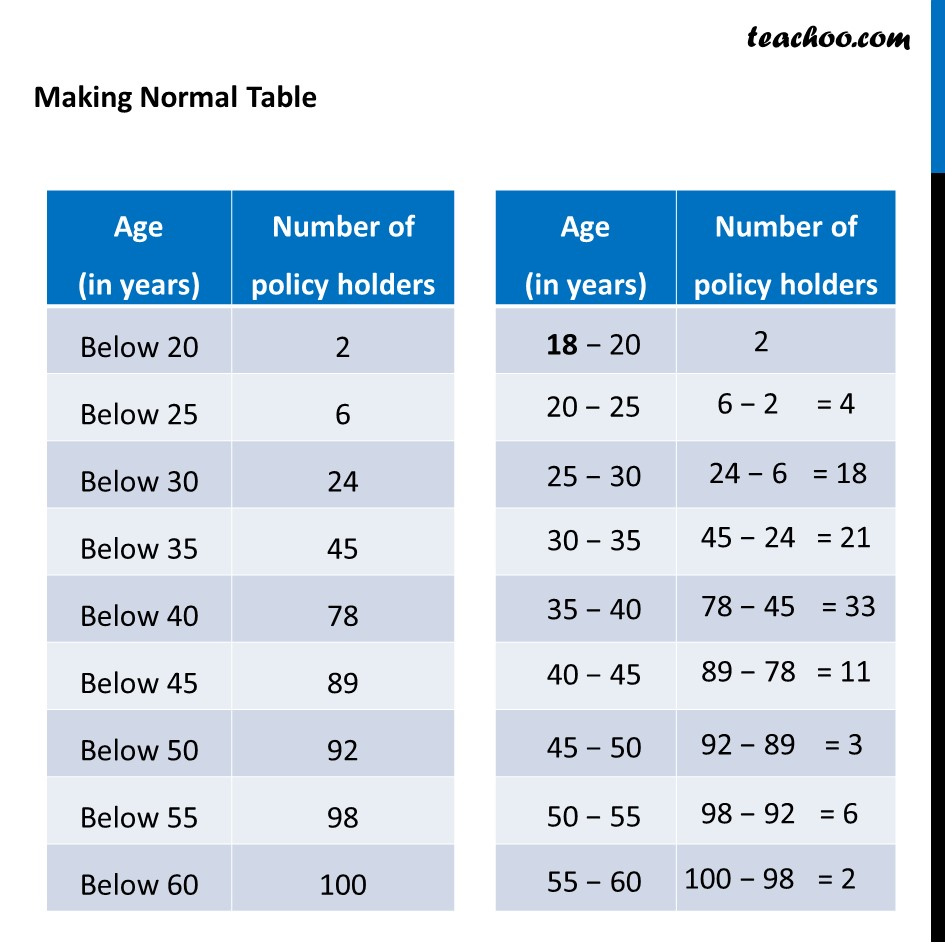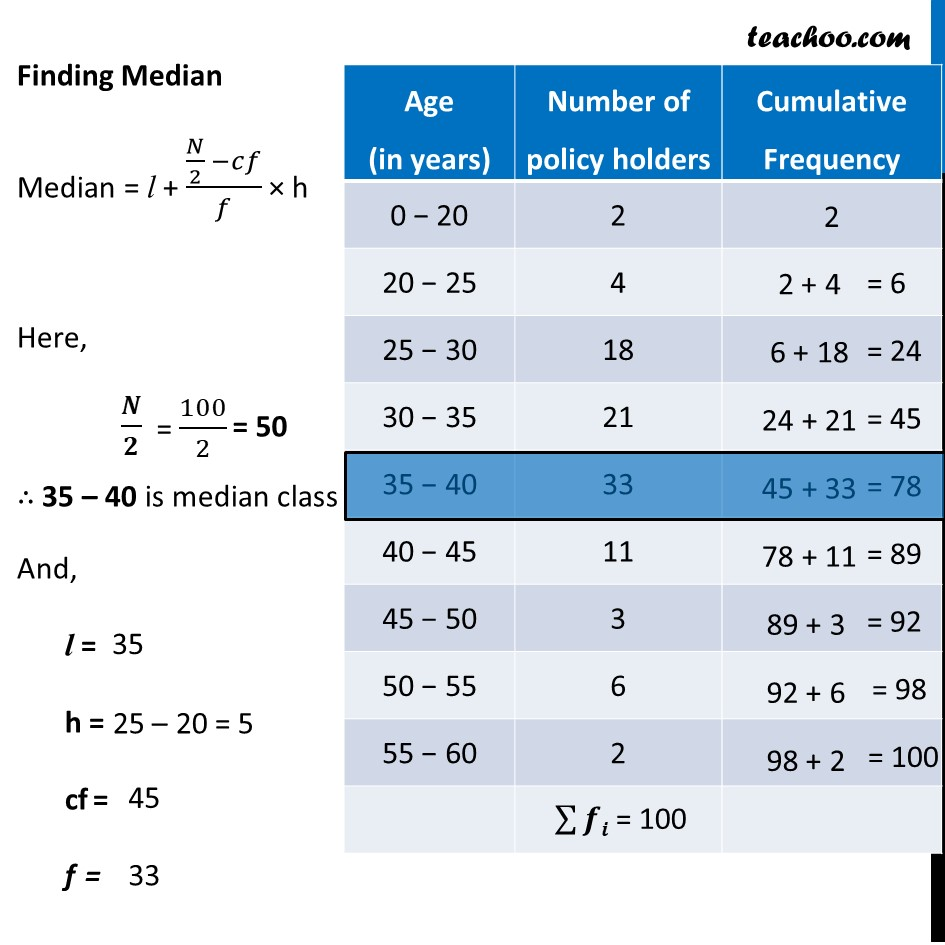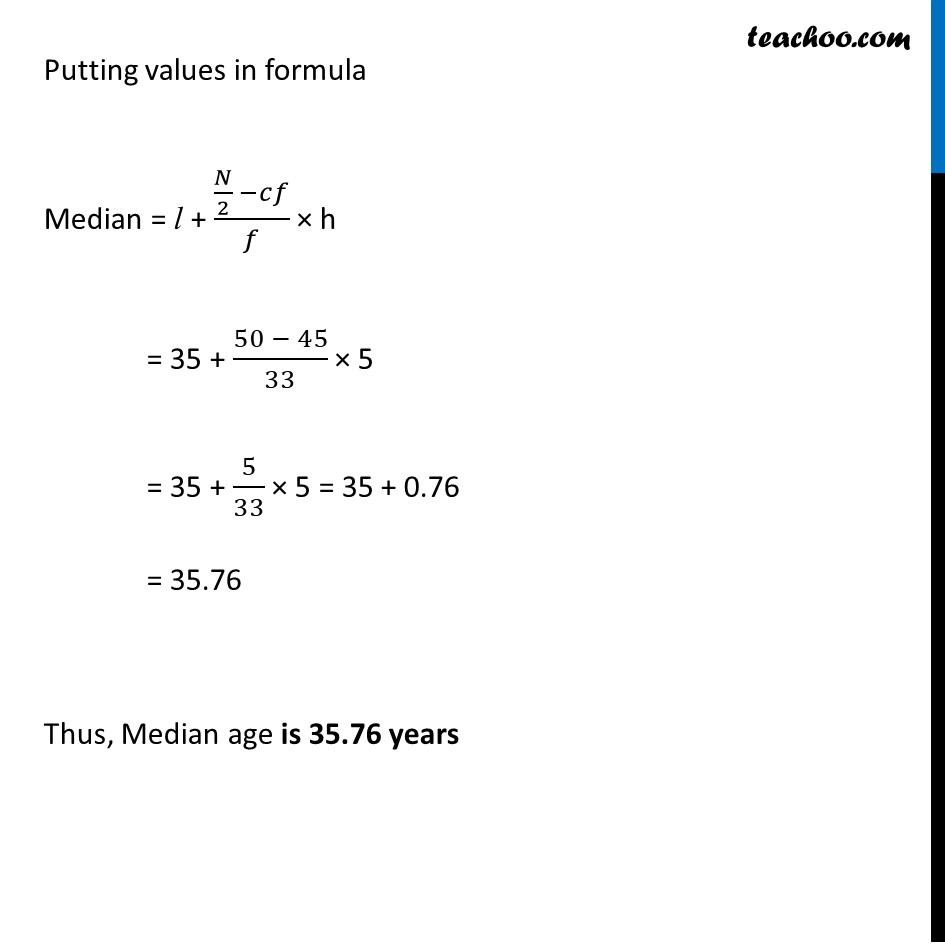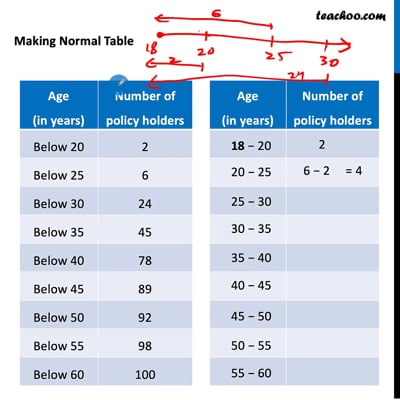This video is only available for Teachoo black users

Maths Crash Course - Live lectures + all videos + Real time Doubt solving!

### Transcript

Ex 14.3, 3 A life insurance agent found the following data for distribution of ages of 100 policy holders. Calculate the median age, if policies are given only to persons having age 18 years onwards but less than 60 year. Making Normal Table Finding Median Median = l + (𝑁/2 −𝑐𝑓)/𝑓 × h Here, 𝑵/𝟐 ∴ 35 – 40 is median class And, l = h = cf = f = Putting values in formula Median = l + (𝑁/2 −𝑐𝑓)/𝑓 × h = 35 + (50 − 45)/33 × 5 = 35 + 5/33 × 5 = 35 + 0.76 = 35.76 Thus, ﻿Median age is 35.76 years